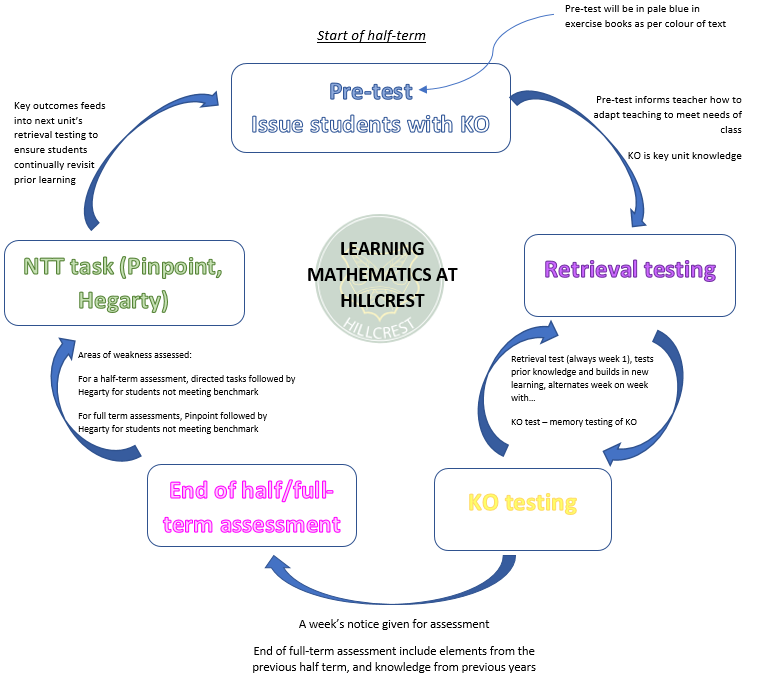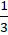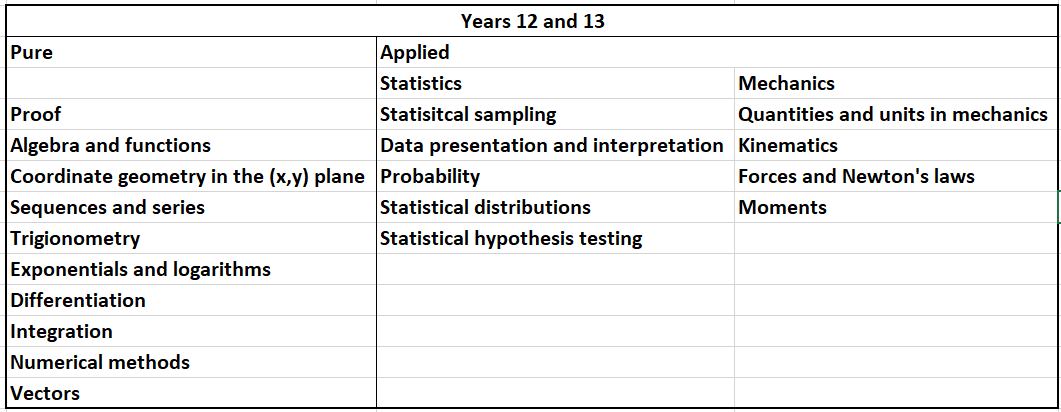••••••# Maths

Mathematics is the language of the universe, learning and exploring it is a creative and satisfying endeavour.

“It is impossible to be a mathematician without being a poet in soul” Sofia Kovalevskaya

## Curriculum Intent

Through effective Teaching and Learning, we deliver and exceed the national curriculum in mathematics. The learning journey is mapped, so students are constantly building and reinforce their mathematical learning, such that they are skilled in both conceptual understanding and application.

Students will be able to succeed in national examinations and take their mathematics to use and apply to both other areas of learning and their wider lives.

Students will be logical, critical thinkers with a natural curiosity around problem-solving.

Learning in mathematics — what it will looks like

Key milestones of Teaching and Learning cycle in mathematics:

i. Pre-testing
ii. Knowledge Organisers (KO’s)
iii. Retrieval testing
iv. KO testing
v. End of term assessment
vi. Now Try This (NTT) assessment follow upThis structure of learning applies for years 7-10. Year 11 follows a similar structure, with allowances for exam preparation. Sixth form learning involves greater elements of extended learning to master mathematical concepts.

## Curricular Journey

The mathematics curriculum at Hillcrest allows students to develop and explore areas of mathematics in a logical and cumulative manner. Interconnection and review of topics if critical to our learning journey and students are allowed plentiful opportunities to apply learnt skills in a variety of contexts.

Key: Number (red), Geometry (orange), Algebra (green), Ratio and Proportion (blue), Probability and Statistics (purple)

Topics of the same colour interconnect, though there is regular overlap between skills.

For example, in term 3a, in year 8, students study angles, which is part of geometry. However, they will require the use of number and algebra skills to support this work.

Key Stage 3 purpose: to establish fluency, reasoning and problem-solving. To explore concepts with clarity and depth

## Year 7

 Term 1a Making generalisations about the number system 1 Number systems and axioms Factors and multiples and order of operations Term 1b Making generalisations about the number system 2 Positive and negative numbers Expressions, equations and sequences Term 2a 2-D Geometry Angles Classifying 2-D shapes Constructing triangles and quadrilaterals Term 2b The Cartesian plane Coordinates Area of 2-D shapes Transforming 2-D figures Term 3a Fractions Primes, factors and multiples Fractions Term 3b Ratio and Proportion Ratio Percentages

## Year 8

 Term 1a Equations and Inequalities Forming and solving equations Forming and solving inequalities Term 1b Graphs Linear graphs Accuracy and estimation Term 2a Proportional reasoning Ratio, real life graphs and rate Direct and inverse proportion Term 2b Representations and reasoning with data Univariate data Bivariate data Term 3a Angles Parallel lines Angles in Polygons Bearings Term 3b Area, volume and surface area Circles and composite shapes Volume of prisms Surface area of prisms

## Year 9

 Term 1a Probability Probability Sample spaces Term 1b Quadratics Quadratic expressions and equations Term 2a Geometry of triangles Construction, congruence and loci Pythagoras' Theorem Term 2b Ratio and Proportion Enlargement and similarity Surds and trigonometry Term 3a Simultaneous Equations Solving graphically Solving algebraically Term 3b Reasoning with number Indices and standard form Growth and decay

Following the Key Stage 3 curriculum, students enter the Higher (grades 4-9) or Foundation (grades 1-5) GCSE pathway.

Key Stage 4 purpose: to build on prior knowledge and acquire advanced skills. To apply knowledge and skills to variety of contexts.

## Year 10

 Year 10 Higher Year 10 Foundation Term 1a Basic number Basic Number Fractions, ratio and proportion Measure and Scale Drawings Statistical diagrams and averages Charts, tables and averages Number and sequences Term 1b Algebra - sequences Angles Ratio, proportion and rates of change Angles Number properties Transformations, constructions and loci Term 2a Algebraic manipulation Approximations Decimals and Fractions Length, area and volume Linear Graphs Term 2b Linear Graphs Expressions and Formulae Right-angled triangles Ratio, speed and proportion Term 3a Similarity Perimeter and Area Transformations Powers and Standard Form Probablility and Events Term 3b Equations and Inequalities Volume and Surface Area Rational numbers, terminating and recurring decimals Linear Equations

## Year 11

 Year 11 Higher Year 11 Foundation Term 1a Accuracy, Indices and Surds Percentages and Compound Measures Percentages and Variation Quadratic Equations Statistical representation and interpretations Term 1b Quadratic Inequalities Constructions and Loci Sampling and more complex diagrams Curved shapes and Pyramids MOCK EXAMS MOCK EXAMS Term 2a Probability - combined events Sequences Properties of a circle Right-angled triangles Term 2b Variation Congruence and Similarity Triangles Probability - combined events Graphs Indices and Standard Form Term 3a Graph transformations Simultaneous Equations Functions Linear Inequalities Non-linear graphs GCSE EXAMINATIONS GCSE EXAMINATIONS

To continue with higher education in any subject, students require a grade 4 or 5 in mathematics. Science A-Levels predominantly require students to attain a grade 6 in mathematics, and to progress onto A-Level mathematics, students must attain at least a grade 7.

## Key Stage 5 (A-Level)

Key Stage 4 purpose: to develop advanced mathematical knowledge and skills and a logical approach to problem-solving.

Overarching elements:

1. Mathematical argument, language and proof

2. Mathematical problem-solving

3. Mathematical modelling

These are explored through a programme of study comprised ofPure mathematics andApplied mathematics (comprising Statistics and Mechanics equally).

All areas are taught in parallel such that the AS-Level qualification can be obtained at the end of year 12. Students complete the full A-Level content in year 13.A-Level mathematics is a ‘facilitating A-Level’ and is useful preparation a wide range of degree courses and is a requirement for STEM and Economics undergraduate study.

Assessment Information

Key Stage 3 – students will have internal half-termly assessments and end of year examinations to help us determine their progress

Key Stage 4 (GCSE) – students will have internal half-termly assessment and end of year/mock examinations to help us determine their progress. They will sit an external examination at the end of year 11 comprising of 3 x 1.5 hours (2 of the papers are calculator examinations) – Exam Board: AQA

Key Stage 5 (A-Level) - students will have internal half-termly assessments to help us determine their progress. They will sit external examinations at the end of year 12 and 13 – Exam Board: EdExcel